# Resolving a Vector into Components

Resolving a Vector into Components

The following figure shows a vector $$\overrightarrow {AB}$$ of length $$5\sqrt 2$$ units, inclined at an angle of 450 to the positive x-direction: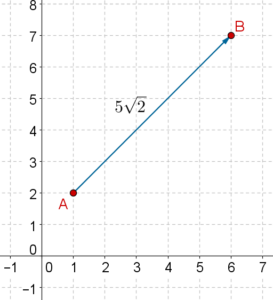Can we express this vector using the $$\hat i$$- $$\hat j$$ system?

Observe the following figure, where we have resolved the vector $$\overrightarrow {AB}$$ into two components $$\overrightarrow {AC}$$ and $$\overrightarrow {CB}$$, where $$\overrightarrow {AC}$$ is parallel to the x-axis, and $$\overrightarrow {CB}$$ is parallel to the y-axis: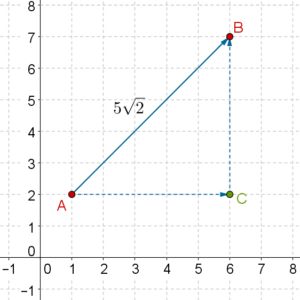We note that:

\begin{align}&\left| {\overrightarrow {AC} } \right| = 5\sqrt 2 \cos {45^0} = 5\sqrt 2 \times \frac{1}{{\sqrt 2 }} = 5\\\,\,\, \qquad\qquad \qquad\qquad &\qquad\qquad\qquad\qquad\;\;\Rightarrow \,\,\,\overrightarrow {AC} = 5\hat i\\&\left| {\overrightarrow {CB} } \right| = 5\sqrt 2 \sin {45^0} = 5\sqrt 2 \times \frac{1}{{\sqrt 2 }} = 5\\\,\,\, \qquad\qquad \qquad \qquad &\qquad\qquad\qquad\qquad\;\Rightarrow \,\,\,\overrightarrow {CB} = 5\hat j\end{align}Thus,

$\overrightarrow {AB} = \overrightarrow {AC} + \overrightarrow {CB} = 5\hat i + 5\hat j$

Let us see another example of resolving a vector into its components (which simply means finding the $$\hat i$$-component and the $$\hat j$$-component). The following figure shows a vector $$\overrightarrow {PQ}$$ of length 6 units inclined at an angle of 1200 to the positive x-direction: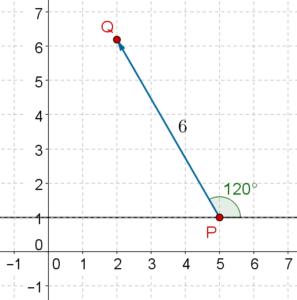What will be the components of $$\overrightarrow {PQ}$$?

Observe the following figure: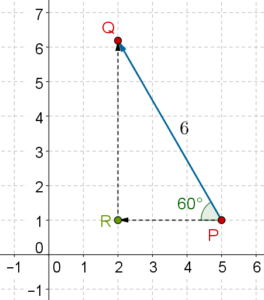$$\overrightarrow {PR}$$ is the $$\hat i$$-component of $$\overrightarrow {PQ}$$, while $$\overrightarrow {RQ}$$ is the $$\hat j$$-component of $$\overrightarrow {PQ}$$. We have:

$\begin{array}{l}\left| {\overrightarrow {PR} } \right| = 6\cos {60^0} = 6\left( {\frac{1}{2}} \right) = 3\\ \qquad \qquad \qquad \quad \Rightarrow \,\,\,\overrightarrow {PR} = - 3\hat i\\\left| {\overrightarrow {RQ} } \right| = 6\sin {60^0} = 6\left( {\frac{{\sqrt 3 }}{2}} \right) = 3\sqrt 3 \\ \qquad \qquad \qquad \quad \Rightarrow \,\,\,\overrightarrow {RQ} = 3\sqrt 3 \hat j\end{array}$

We can now specify $$\overrightarrow {PQ}$$ using the $$\hat i$$- $$\hat j$$system:

$\overrightarrow {PQ} = \overrightarrow {PR} + \overrightarrow {RQ} = - 3\hat i + 3\sqrt 3 \hat j$

The technique of resolving a vector into its components is extensively used in the study of Physics, and must be practiced as much as possible.

Example 1: A vector is inclined at an angle of $$- {30^0}$$ to the positive x-direction, and has a length of 10 units. Specify the vector using the $$\hat i$$- $$\hat j$$system.

Solution: Consider the following figure: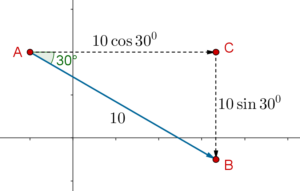We have:

$\begin{array}{l}\left| {\overrightarrow {AC} } \right| = 10\cos {30^0} = 10\left( {\frac{{\sqrt 3 }}{2}} \right) = 5\sqrt 3 \\\left| {\overrightarrow {CB} } \right| = 10\sin {30^0} = 10\left( {\frac{1}{2}} \right) = 5\end{array}$

Thus,

$\overrightarrow {AB} = \overrightarrow {AC} + \overrightarrow {CB} = 5\sqrt 3 \widehat i - 5\widehat j$

Example 2: A force of magnitude F is acting on a box at an angle of $$\theta$$to the horizontal. Take the horizontal right direction as the $$\widehat i$$ direction, and the vertical up direction as the $$\widehat j$$ direction. Specify the force $$\overrightarrow F$$ using this $$\widehat i$$- $$\widehat j$$ system.

Solution: The vector components of the force $$\overrightarrow F$$ are shown below: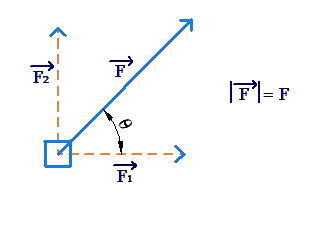We have:

$\overrightarrow {{F_1}} = F\cos \theta \widehat i,\,\,\,\overrightarrow {{F_2}} = F\sin \theta \widehat j$

Thus,

$\overrightarrow F = \overrightarrow {{F_1}} + \overrightarrow {{F_2}} = F\cos \theta \widehat i + F\sin \theta \widehat j$

Example 3: A vector $$\overrightarrow a$$ is specified in the $$\widehat i$$- $$\widehat j$$ system as

$\overrightarrow a = 3\widehat i - 5\widehat j$

Find the magnitude of $$\overrightarrow a$$, and its inclination with the positive x-direction.

Solution: The following figure shows the vector $$\overrightarrow a$$: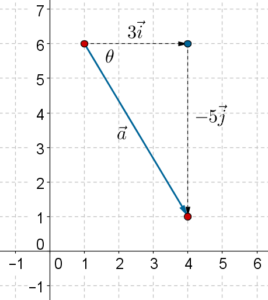Using the Pythagoras Theorem, we have:

$\left| {\overrightarrow a } \right| = \sqrt {{3^2} + {5^2}} = \sqrt {34}$

The angle $$\theta$$ is given by

$\tan \theta = \frac{5}{3}\,\,\, \Rightarrow \,\,\,\theta = {\tan ^{ - 1}}\left( {\frac{5}{3}} \right)$

Thus, the angle of inclination of this vector with the horizontal is $$- {\tan ^{ - 1}}\left( {\frac{5}{3}} \right)$$.

We note that in general, a vector $$\overrightarrow r = x\widehat i + y\widehat j$$ will have a magnitude of $$\left| {\overrightarrow r } \right| = \sqrt {{x^2} + {y^2}}$$.

Download Vectors Worksheets
Vectors
grade 10 | Questions Set 1
Vectors
grade 10 | Answers Set 1
Vectors
grade 10 | Questions Set 2
Vectors
grade 10 | Answers Set 2
More Important Topics
Numbers
Algebra
Geometry
Measurement
Money
Data
Trigonometry
Calculus